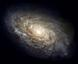Astronomy 162: Introduction to Stars, Galaxies, & the Universe Prof. Richard Pogge, MTWThF 9:30

# Lecture 22: The Cosmic Distance Problem

## Key Ideas

Geometric Distances
Trigonometric Parallaxes

Luminosity Distances
"Standard Candles"
Spectroscopic Parallaxes
Cepheid Variables
RR Lyrae Variables

## The Distance Problem

Measuring accurate distances remains the biggest technical problem in Astronomy.

Distances are necessary for estimating:

• The total energy released by objects (Luminosity)
• The physical sizes of objects
• The masses of objects
• The distribution of objects in space

## Geometric Distances

Direct measurements of distances using geometry.

Solar System Distances:

• Orbit Geometry (Copernicus)

Stellar Distances: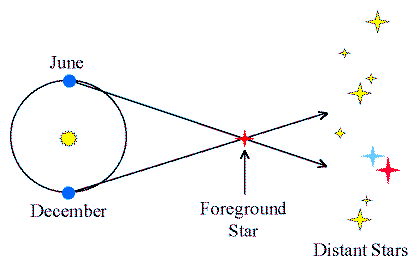(Click on the image to view at full scale [Size: 7Kb])

## Parallax Limits

Ground-based parallaxes are measured to ~0.01-arcsec
• good distances out to 100 pc
• < 1000 stars this close

Hipparcos satellite measures parallaxes to ~0.001-arcsec

• good distances out to 1000 pc
• ~100,000 stars

## Luminosity Distances

Indirect distance estimate:
• Measure the object's Apparent Brightness, B
• Assume the object's Luminosity, L
• Solve for the object's Luminosity Distance, dL, by applying the Inverse Square Law of BrightnessWe call this the Luminosity Distance (dL) to distinguish it from distances estimated by other means (e.g. geometric distances from parallaxes).

The only observable is the object's Apparent Brightness, B. The missing piece is the luminosity, (L), which must be inferred in some way.

## Standard Candles

Any object whose Luminosity you know ahead of time ("a priori") is known as a Standard Candle.

The way you establish that a class of objects is a standard candle is via a multi-step calibration procedure known as the "Bootstrap Method".

Bootstrap Method:

• Calibrate the Luminosities of nearby objects for which you have a Trigonometric Parallax distance.

• Identify distant but similar objects, using a distance-independent property that they share.

• Assume that the distant objects have the same Luminosity as the nearby objects for which you have distances.
Once you have a calibration of a set of standard candles, you can then apply them to measuring distances to objects that are too far away for geometric methods like parallaxes.

## Spectroscopic "Parallaxes"

Distance-Independent Property:
• The observed spectrum of the star.

Physics:

• The Spectral Type (OBAFGKML) tells you the star's Temperature.
• The Luminosity Class (I..V) tells you which region of the H-R Diagram the star belongs in.
• Together, they give the star a unique location on a calibrated H-R Diagram.

Method:

• Build up a calibrated H-R Diagram for nearby stars with good parallax distances.
• Get Spectral Type & Luminosity Class of the distant star from its spectrum.
• Locate the star in the calibrated H-R Diagram
• Compute the Luminosity Distance (dL) from is measured Apparent Brightness.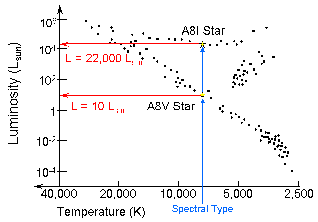NOTE: Despite the name, the method has nothing to do with measuring geometric "parallaxes".

Distance Limit:

• Practical limit few 100,000 pc.
• Works best for star clusters.

Problems:

• Luminosity Classes are only roughly defined.
• H-R diagram location depends on composition.
• Faint spectra give poor classifications.
While somewhat difficult to use accurately for individual stars, the method of spectroscopic parallaxes works best for clusters of stars where you can average over many measurements.

## Periodic Variable Stars

Stars whose brightness varies regularly with a characteristic, periodic (repeating) pattern.

Distance-Independent Property:

• Period (repetition time) of their cycle of brightness variations.

Physics:

• Period-Luminosity Relations exist for certain classes of periodic variable stars.
• Measuring the Period gives the Luminosity.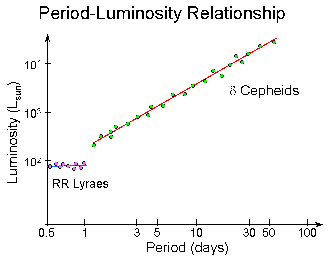## Cepheid Variables

Class of rhythmically pulsating Supergiant stars:
• Found in young star clusters
• Luminosity of ~ 103-4 Lsun
• Brightness Range: few percent to a factor 2-3 in brightness
• Period Range: 1 day up to ~50 days.

Period-Luminosity Relation:

• Longer Period = Higher Luminosity
• P = 3 days, L ~ 103 Lsun
• P = 30 days, L ~ 104 Lsun

Method:

• Measure the pulsation period (P)
• Using the P-L relation, read off the Luminosity (L)
• Compute the Luminosity Distance (dL) from the Apparent Brightness and inferred Luminosity.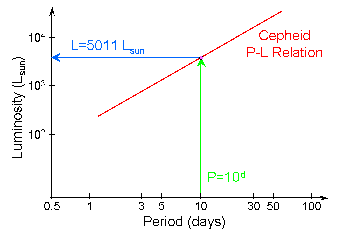Distance Limit:

• 30-40 Megaparsecs (Hubble Space Telescope)
• Crucial for measuring distances to galaxies.

Problems:

• No Cepheids with precise parallaxes (a few now have low-quality parallaxes measured by Hipparcos, but are just at the edge of what Hipparcos can do).
• Two types of Cepheids with different P-L relations (delta Cephei and W Virginis stars, respectively).
Despite the limitations and problems, Cepheid Variable Stars (specifically delta Cephei stars) are one of the most important Standard Candles we use to measure cosmic distances.

## RR Lyrae Variables

Rhythmically pulsating Horizontal-Branch stars:
• Found in old clusters, Galactic bulge & halo
• Luminosity of ~50 Lsun
• Brightness Range: factor of ~ 2-3
• Period Range: few hours to ~ 1 day.
• Relatives of Cepheid Variables

Period-Luminosity Relation:

• Less strong than for Cepheids

Method:

• Same as for Cepheids, but using the RR Lyrae P-L Relation to get an estimate of the Luminosity.

Distance Limit:

• ~1 Megaparsec (Hubble)
• Limited to our Galaxy & Andromeda

Problems:

• No RR Lyrae stars with precision parallaxes
• RR Lyrae stars are fainter than Cepheids, so they are only useful as standard candles relatively nearby.

## The Cosmic Distance Scale

No single method will provide distances on all cosmic scales. Instead, we have to rely on a multi-step approach that is carefully calibrated at each step.

This makes the Cosmic Distance Scale look like a ladder with a series of steps going from near to far:

• Calibrate Parallaxes using the Astronomical Unit (orbit of the Earth)
• Calibrate H-R diagram methods using stars with measured Parallaxes.
• Calibrate Cepheid and RR Lyrae star distances using H-R diagrams.
Inaccuracy and imprecision at each step carries forward into the next, making each subsequent step less accurate.

Part of the challenge is to understand the sources of these inaccuracies and taking them into account.

Return to [ Unit 4 Index | Astronomy 162 Main Page ]
Updated: 2006 February 5